## Pandas 101 : Learn Pandas in 10 minutes

Pandas is a popular Python library for data analysis and manipulation. It provides a fast and easy way to work with tabular data, such as CSV files, Excel spreadsheets, or SQL databases.

In this blog post, I will share some useful pandas cheat sheet that covers some of the most common tasks and operations that you may encounter in your data science projects. Whether you need to filter, sort, group, summarise, understand the data, pandas has a function or method for you. Let’s get started!

You can find the jupyter notebook for this post here : Click here

# Install Pandas

You can install pandas in two ways:

## Using pip

``````pip install pandas
``````

## Using conda

``````conda install -c anaconda pandas
``````

# Import Pandas

``````import pandas as pd
``````

The data can be one-dimensional data or multi-dimensional data. First, I will give you an overview of one-dimensional data, then we’ll dive deep into multi-dimensional data.

# Series

A pandas series is a one-dimensional array of data that can store any type of values, such as numbers, strings, or boolean. You can create a pandas series using `Series()` from a list, a dictionary, or a numpy array.

For example, you can create a series like this:

``````# creating an array using list
temps_list = [1, 2, 3, 4, 5]

# creating a dictionary
temps_dict = {1: 10, 2: 20, 3: 30, 4: 40, 5: 50}

# creating a numpy array
temps_numpy = np.array([100, 200, 300, 400, 500])

# creating a series from list
list_series = pd.Series(temps_list)

# creating a series from dictionary
dict_series = pd.Series(temps_dict)

# creating a series from numpy array
numpy_series = pd.Series(temps_numpy)

list_series
# ------------------- OUTPUT --------------- #
0    1
1    2
2    3
3    4
4    5
dtype: int64
# ------------------- OUTPUT --------------- #

dict_series
# ------------------- OUTPUT --------------- #
1    10
2    20
3    30
4    40
5    50
dtype: int64
# ------------------- OUTPUT --------------- #

numpy_series
# ------------------- OUTPUT --------------- #
0    100
1    200
2    300
3    400
4    500
dtype: int64
# ------------------- OUTPUT --------------- #
``````

You can clearly see that this is an one-dimensional data.

Now, let’s learn about multi-dimensional data.

# DataFrame

A pandas dataframe is a data structure that allows you to store and manipulate tabular data in Python. It is similar to a spreadsheet or a database table, but with more features and flexibility.

You can create a dataframe from various sources, such as lists, dictionaries, files, or web pages. A dataframe has rows and columns, each with a label. You can access and modify the data in a dataframe using various methods and attributes.

For example, you can create a dataframe of names and ages of people like this:

``````# creating a dictionary having names and ages
names_ages_dict = {
"Name": ["Alice", "Bob", "John", "Doe"],
"Age": [18, 24, 35, 11]
}

# creating dataframe from that dictionary
dict_dataframe = pd.DataFrame(names_ages_dict)

dict_dataframe
# --------------------- OUTPUT -------------- #
Name   Age
0 Alice   18
1 Bob     24
2 John    35
3 Doe     11
# --------------------- OUTPUT -------------- #
``````

This is the easiest way to create a dataframe as you can see the keys of dictionary will become column names and the values of the dictionary will be the values of the respective column.

``````# read_csv(file_path).
``````

# Look At The Data

## Look At The Entire Data

``````data
``````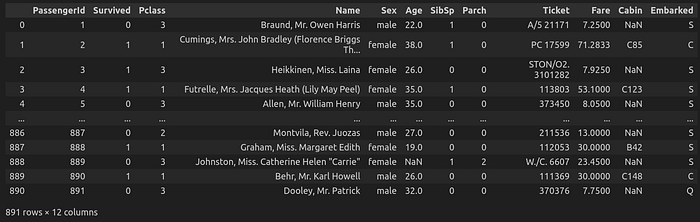## Look At The Top n Rows

You can use `head()`method that takes an optional parameter `n`. `n` denotes the number of rows you want. By default, `n = 5`.

``````# this will output the top 5 rows as by default n = 5
``````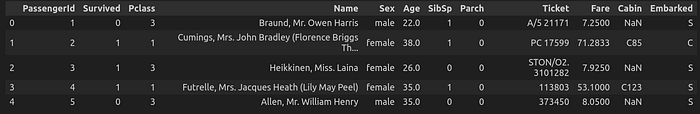``````# this will output the top 10 rows as I've set n = 10
``````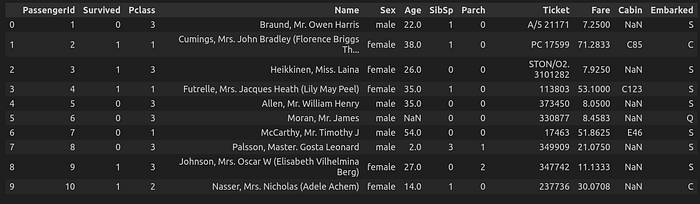## Look At The Bottom n Rows

You can use tail`()`method that takes an optional parameter `n`. `n` denotes the number of rows you want. By default, `n = 5`.

``````# this will output the bottom 5 rows as by default n = 5
data.tail()
``````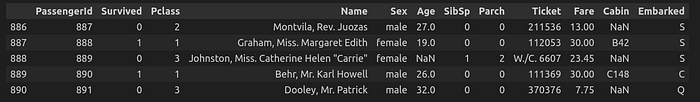``````# this will output the bottom 10 rows as I've set n = 10
data.tail(n=10)
``````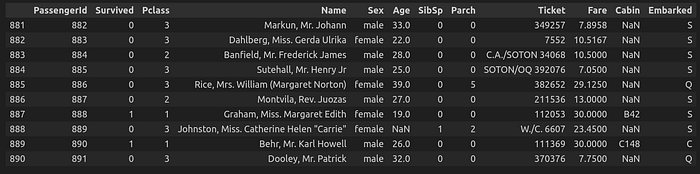# Mathematical Functions on Pandas

## To get the min value

To get the minimum values for every column.

``````data.min()

# ---------------------- OUTPUT --------------------------------- #
PassengerId                      1
Survived                         0
Pclass                           1
Name           Abbing, Mr. Anthony
Sex                         female
Age                           0.42
SibSp                            0
Parch                            0
Ticket                      110152
Fare                           0.0
dtype: object
# ---------------------- OUTPUT --------------------------------- #
``````

## To get the max value

To get the maximum values for every column.

``````data.max()

# ---------------------- OUTPUT --------------------------------- #
PassengerId                            891
Survived                                 1
Pclass                                   3
Name           van Melkebeke, Mr. Philemon
Sex                                   male
Age                                   80.0
SibSp                                    8
Parch                                    6
Ticket                           WE/P 5735
Fare                              512.3292
dtype: object
# ---------------------- OUTPUT --------------------------------- #
``````

## To get the mean value

To get the mean value for every column.

``````data.mean()
# ---------------------- OUTPUT --------------------------------- #
PassengerId    446.000000
Survived         0.383838
Pclass           2.308642
Age             29.699118
SibSp            0.523008
Parch            0.381594
Fare            32.204208
dtype: float64
# ---------------------- OUTPUT --------------------------------- #
``````

## To get the median value

To get the median value for every column.

``````data.median()
# ---------------------- OUTPUT --------------------------------- #
PassengerId    446.0000
Survived         0.0000
Pclass           3.0000
Age             28.0000
SibSp            0.0000
Parch            0.0000
Fare            14.4542
dtype: float64
# ---------------------- OUTPUT --------------------------------- #
``````

## To get the standard deviation

To get the standard deviation value for every column.

``````data.std()
# ---------------------- OUTPUT --------------------------------- #
PassengerId    257.353842
Survived         0.486592
Pclass           0.836071
Age             14.526497
SibSp            1.102743
Parch            0.806057
Fare            49.693429
dtype: float64
# ---------------------- OUTPUT --------------------------------- #
``````

# Accessing Rows and Columns of DataFrame

Now, we’ll learn about how to read every column or every row or a specific column and a specific row. But first, let’s learn about `loc[]` and `iloc[]`.

One of the features of pandas is that it allows us to select and modify data using labels or indices. The `loc[]` and `iloc[]` methods are two ways of doing this. The `loc[]` method selects data based on labels, such as column names or row names. The `iloc[]` method selects data based on integer indices, such as the position of the rows or columns.

Here, I will explain the difference between pandas `loc[]` and `iloc[]` methods, which are used to select data from a DataFrame in Python. I will also show some examples of how to use them.

`loc[]` and `iloc[]` are both indexers, which means they allow us to access specific rows and columns of a DataFrame by using labels or positions. However, they have different ways of doing so.

## loc Method

`loc[]` is a label-based indexer, which means it selects data based on the row and column labels. For example, if we want to select the row with index 0 and the column with label “Name”, we can use:

``````# This will return the Name of first row
data.loc[0, "Name"]

# ------------------------- OUTPUT ----------------------- #
'Braund, Mr. Owen Harris'
# ------------------------- OUTPUT ----------------------- #
``````

`loc[]` also supports slicing. For example, if we want to select “Name” and “Age” of all rows :

``````data.loc[:,["Name", "Age"]]
``````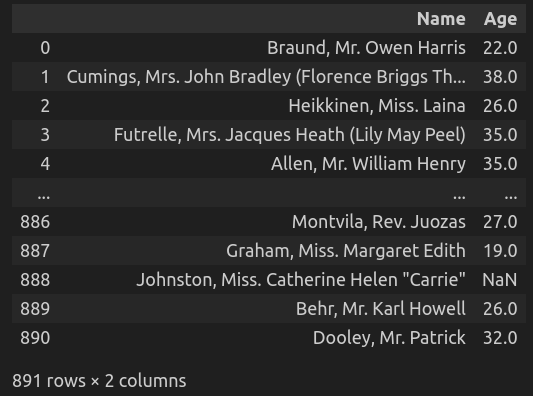## iloc Method

`iloc[]`is a position-based indexer, which means it selects data based on the row and column positions. For example, if we want to select the first row and the fourth column of a DataFrame, we can use:

``````# this will return the Name of the first row as Name column is in position = 3
data.iloc[0, 3]

# ---------------------- OUTPUT ---------------------- #
'Braund, Mr. Owen Harris'
# ---------------------- OUTPUT ---------------------- #
``````

`iloc[]` also supports slicing. For example, if we want to select the first three rows and the Name, Sex and Age columns of a DataFrame, we can use:

``````data.iloc[0:3, 3:6]
``````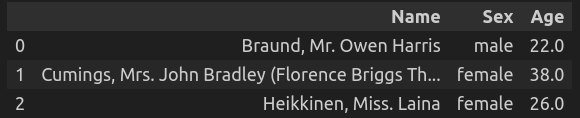## Differences Between loc and iloc

One important difference between `loc[]` and `iloc[]` is that `loc[]` includes the last element of the slice, while `iloc[]` excludes it. For example, if we want to select the first two rows of a DataFrame, we can use:

``````data.loc[0:1] # includes both rows 0 and 1
data.iloc[0:1] # includes only row 0
``````

Another difference is that `loc[]` can accept boolean arrays as inputs, while `iloc[]` cannot. For example, if we want to select all rows where the ‘Age’ column is greater than 18, we can use:

``````data.loc[data["Age"] == 18] # works fine
data.iloc[data["Age"] == 18] # raises an error
``````

To summarize, `loc[]`and `iloc[]` are both useful methods for selecting data from a DataFrame in Python. `loc[]` is based on labels, while `iloc[]`is based on positions. `loc[]` includes the last element of the slice, while `iloc[]` excludes it. `loc[]` can accept boolean arrays, while `iloc[]` cannot.

# Describe and Info Of The Data

We will explore two useful methods that can help us understand the basic properties and statistics of our data: describe and info.

The describe method returns a summary of the numerical columns in a DataFrame or a Series. It calculates some common descriptive statistics, such as count, mean, standard deviation, minimum, maximum and percentiles.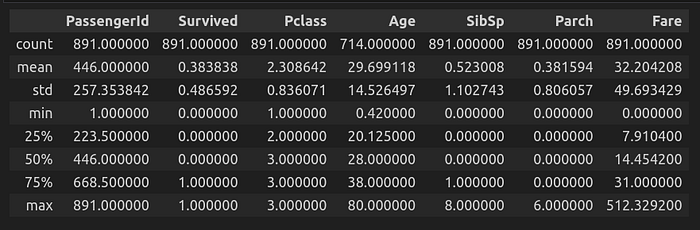To make sense of the above output, the output says that average or mean age of passenger is 29. Another observation can be that the maximum fare is 512.

This way you can make sense of the output of `describe()` method.

## Info

The info method returns a concise summary of the non-numerical columns in a DataFrame or a Series. It shows the index dtype and column dtypes, non-null values and memory usage.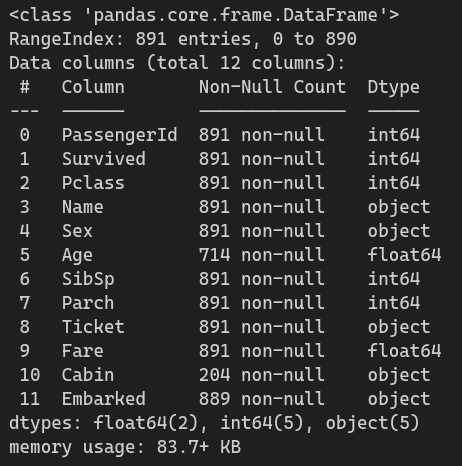You can see that, at-first the output show that it’s a range-index and it has 891 entries. Then it shows that there are 12 columns and then it shows those 12 columns position, name, non-null count, and data type.

# Sorting The Data

We can sort the data in multiple ways:

## Sort Using A Single Column

Let say you want to sort the data by ages in ascending order. By default, the data will be sorted in ascending order.

``````# sorting the data by fare in ascending order
data.sort_values("Fare")
``````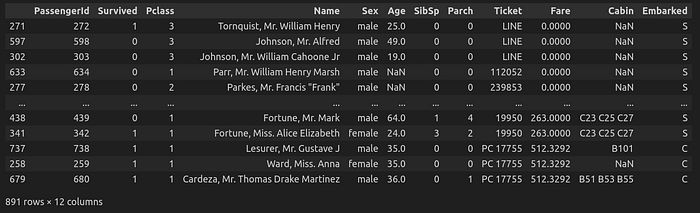``````# sorting the data by faire in descending order
data.sort_values("Fare", ascending=False)
``````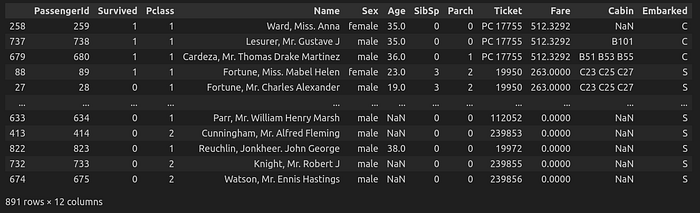## Sort Using Multiple Columns

``````data.sort_values(["Fare", "Pclass"])
``````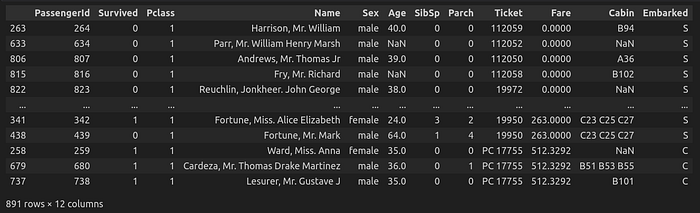``````data.sort_values(["SibSp", "Pclass"], ascending=False)
``````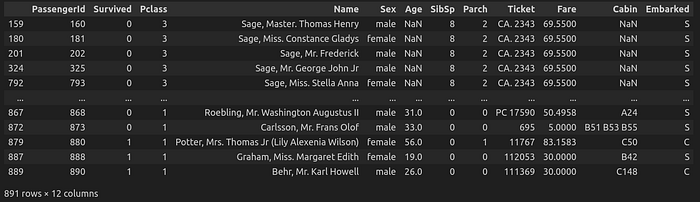We can also sort different column in their own way.

``````# This will sort the Pclass is ascending order and Age is descending order
data.sort_values(["Pclass", "Age"], ascending=[True, False])
``````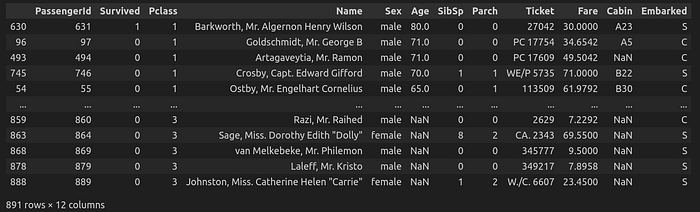# Manipulating The Data

``````# creating a new column named total -> sum of SibSp and Parch
data["Total"] = data.iloc[:,[6,7]].sum(axis=1)
data
``````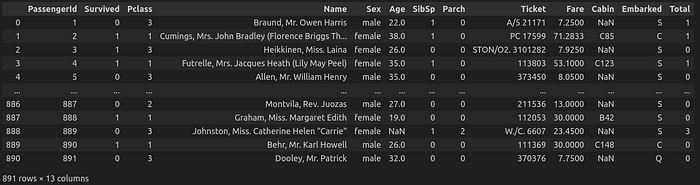## Deleting A Column

``````# drop a column
data.drop(columns=["Total"], inplace=True)
``````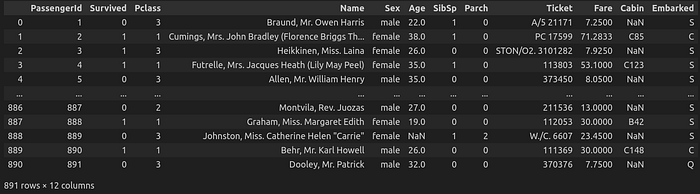## Reordering The Columns

Let say you want to move the “Fare” column to the right most position

``````# reorder the columns using loc
data = data.loc[:,["PassengerId","Survived","Pclass", "Name", "Sex", "Age", "SibSp", "Parch", "Ticket", "Cabin", "Embarked", "Fare"]]
data

# reorder the columns using iloc
data = data.iloc[:,[0,1,2,3,4,5,7,8,10,11,9]]
data
``````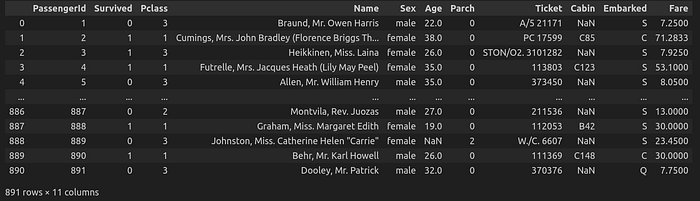# Filtering Data

``````# get only passenger's having Pclass == 1
data.loc[data["Pclass"] == 1]
``````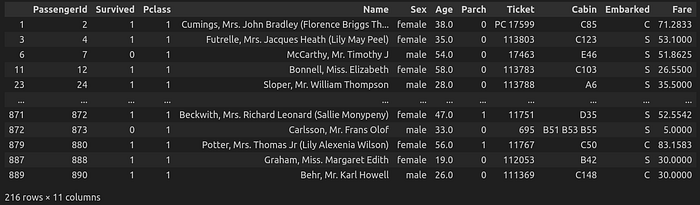``````# getting only passenger's having Pclass == 1 and survived
new_data = data.loc[(data["Pclass"] == 1) & (data["Survived"] == 1)]
new_data = new_data.reset_index(drop=True)
new_data
``````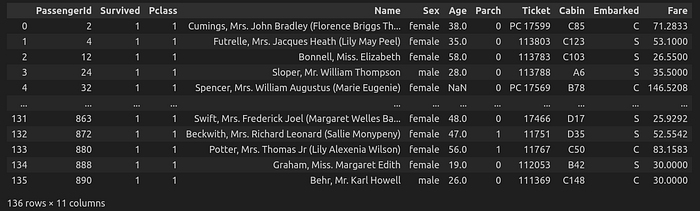# Conditional Change

``````# replace the sex value of male to 1
data.loc[data["Sex"] == "male", "Sex"] = 1
data.loc[data["Sex"] == "female", "Sex"] = 0
data
``````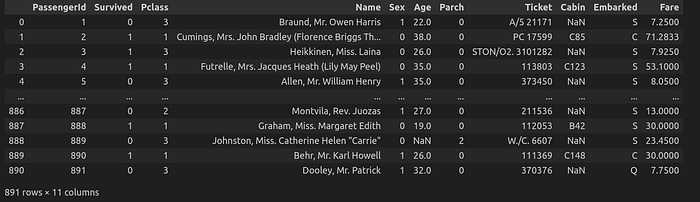# Aggregation Using GroupBy

May be you have tried to learn about groupby in the past and got confused but don’t worry, here I’ll give you enough examples to get comfortable with groupby. So, let’s start!!!

Let say you want to know how many females and males were present in the ship.

Here, we will use the groupby method on the column “Sex” and then use the function count, so that it’ll count the respective column values.

``````data.groupby("Sex").size()

# ------------------------- OUTPUT ------------------ #
Sex
0    314
1    577
dtype: int64
# ------------------------- OUTPUT ------------------ #
``````

Let say now you want to find how many male and female passengers survived.

``````data.groupby(["Sex", "Survived"]).size()

# ------------------------ OUTPUT ----------------------- #
Sex  Survived
0    0            81
1           233
1    0           468
1           109
dtype: int64

# ------------------------ OUTPUT ----------------------- #
``````

Let say you want to find number of male and female passengers for each embark and their min,max, and mean age.

``````data.groupby(["Sex","Embarked","Pclass"]).agg({'PassengerId' : 'count' , 'Age':['min','max','mean']})
``````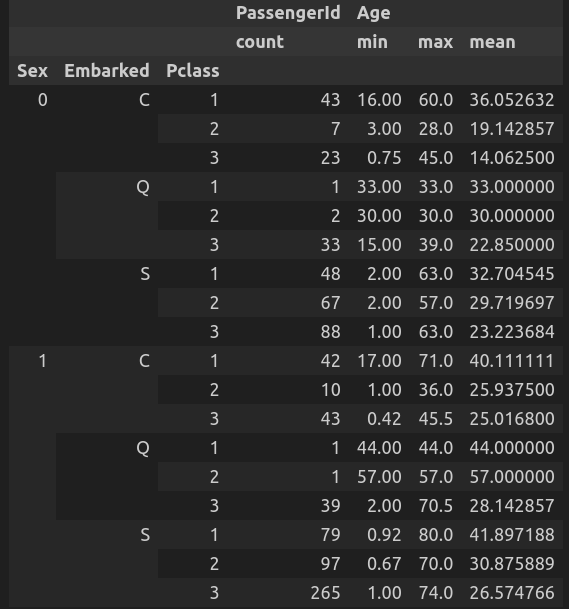# Handling Missing Values

## Get Number of null values in each column

``````data.isnull().sum()
# -------------------------- OUTPUT --------------------------- #
PassengerId      0
Survived         0
Pclass           0
Name             0
Sex              0
Age            177
SibSp            0
Parch            0
Ticket           0
Fare             0
Cabin          687
Embarked         2
dtype: int64
# -------------------------- OUTPUT --------------------------- #
``````

This will return the total number of null or missing values in every column.

Note: If you don’t use .sum(), then it’ll return a dataframe consisting of Boolean values where a `True` means it is null and a `False` means it is not null.

``````data.isnull()
``````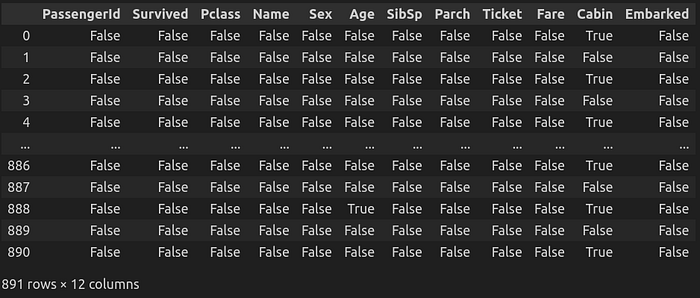To handle the missing values in a dataset, we can do one of the two things:

• Drop the columns which has missing values

• Fill the missing values with some value

## Dropping Columns

You have already learnt how to drop specific columns. Here, I’ll share a strategy with you about when you should drop a column.

If a column has more null values than non-null values, then drop the column.

## Filling Missing Values of a Column

There are multiple ways of filling the missing values of a column:

• You can simply hard code a specific value, which will replace all the null values.

• You can also fill the null values with the mean,median or mode of the column values.

In the above image, you saw that there are 177 missing values in `Age` column. So. let’s fill the null values with the average age of all the passengers.

``````average_age_of_passenger = data["Age"].mean()
data.loc[:,"Age"].fillna(average_age_of_passenger, inplace=True)

data["Age"].isnull().sum() # this will show the number of missing values in Age

# ------------------------ OUTPUT ----------------------- #
0
# ------------------------ OUTPUT ----------------------- #
``````

As you can see the missing values are now replaced with the mean age of passengers.

I can’t show you all the different ways to fill missing values, but in your data science journey, you’ll learn a lot of advanced methods to handle missing values.

# Miscellaneous

## value_counts() method

You can use the `value_counts()` function to count the frequency of unique values in a pandas Series or a Dataframe column.

To get the total number of male and female passengers:

`````` data["Sex"].value_counts()
# -------------------------- OUTPUT --------------------------- #
male      577
female    314
Name: Sex, dtype: int64
# -------------------------- OUTPUT --------------------------- #
``````

## Conclusion

In this blog post, we have learned the basics of pandas, a powerful Python library for data analysis and manipulation. We have seen how to create and manipulate data frames, how to perform common operations such as filtering, sorting, grouping and aggregating.

I hope you have enjoyed this pandas 101 blog post and learned something new. If you have any questions or feedback, please leave a comment below. Thank you for reading!